Smartick is an advanced online program that teaches kids math and coding in only 15 min. a day

Dec01

# Using Mixed Numbers to Represent Improper Fractions

In today’s post we’re going to learn about mixed numbers, which are much easier to interpret than the improper fractions they represent.

We’re going to look at some examples of the exercises and problems that you can work on in Smartick and the types of numbers that you can work on in these activities. You’ll be able to do them almost without thinking!

### Improper fractions as a mixed number

Remember that a mixed number is a numerical way of representing a fraction greater than a unit (improper fraction), or in other words, to represent fractions in which the numerator is greater than the denominator.

• If you look at the improper fraction 6713, it might not be easy to visualize the number that it represents, since it is greater than the unit (because the numerator is greater than the denominator, 67 > 13).
• But if you convert it into a mixed number, you can understand the number it represents much more easily.
• To do this, the first thing you need to do is divide the numerator of the fraction by the denominator, to find out how many whole units the number contains.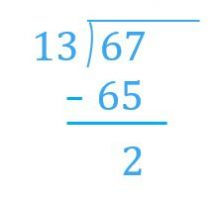• Since 65 = 13 x 5, we can separate the 67 into 65 and 2, and one of the parts will be divisible by 13 and the other won’t: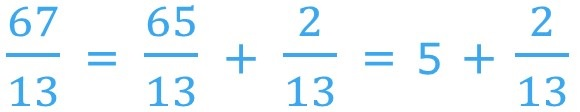• Now, it’s really easy to write the mixed number: first we write the whole number and then the fraction that’s smaller than the unit: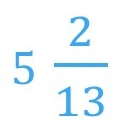• Using this representation it’s easy to see that the number is made up of 5 whole units and 2 thirteenths of another unit.

Now we’re going to look at some examples of the exercises and problems involving mixed numbers that you can work on in Smartick.

### Example 1: Mixed numbers exercise

In the first type of exercise, we can practice writing the mixed number from its representation with pie charts. As you can see, this example shows a completely colored circle, and 1 equal part out of 4 of another circle colored.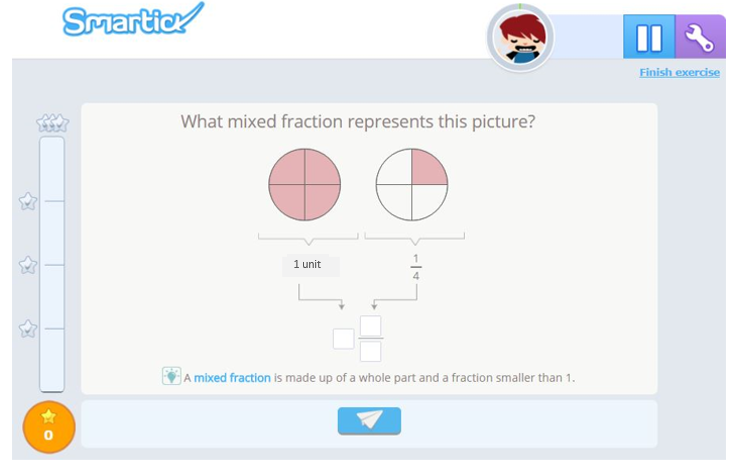Therefore, it’s easy to see that the number represented graphically is 114: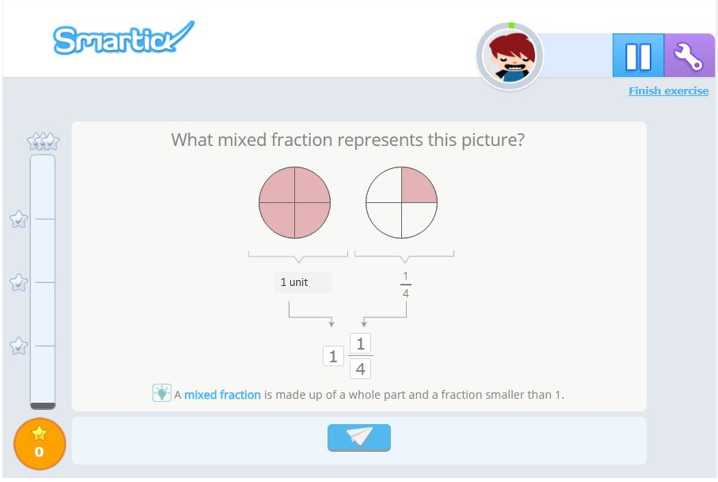### Example 2: Mixed numbers exercise

In the second type of exercise we’re going to use pizzas to help us practice converting an improper fraction into a mixed number. As you can see in the example, we have 2 whole pizzas and three-fourths of another pizza.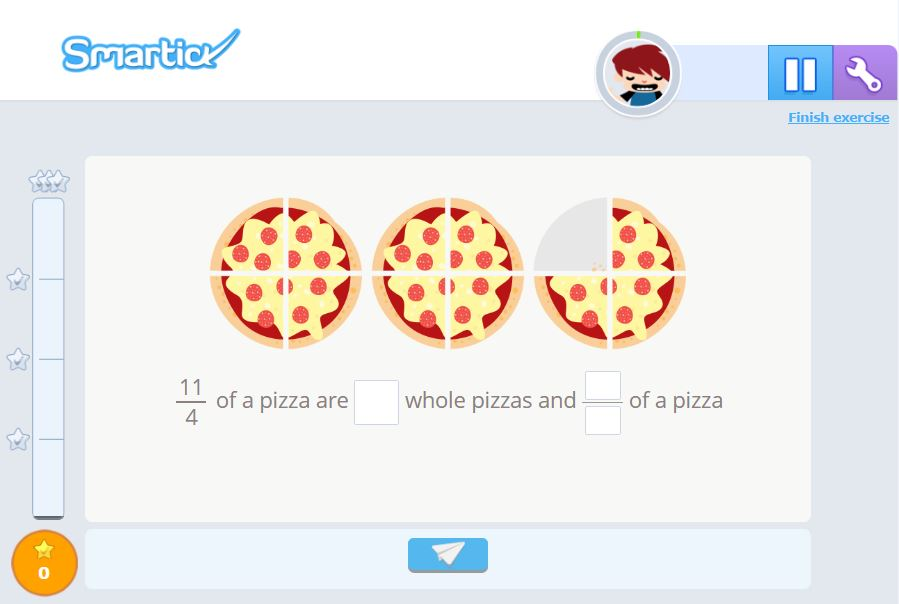You can easily see that 114 of pizza is 2 whole pizzas and 34 of another pizza. In other words, the mixed number that corresponds to the improper fraction 114 is 234.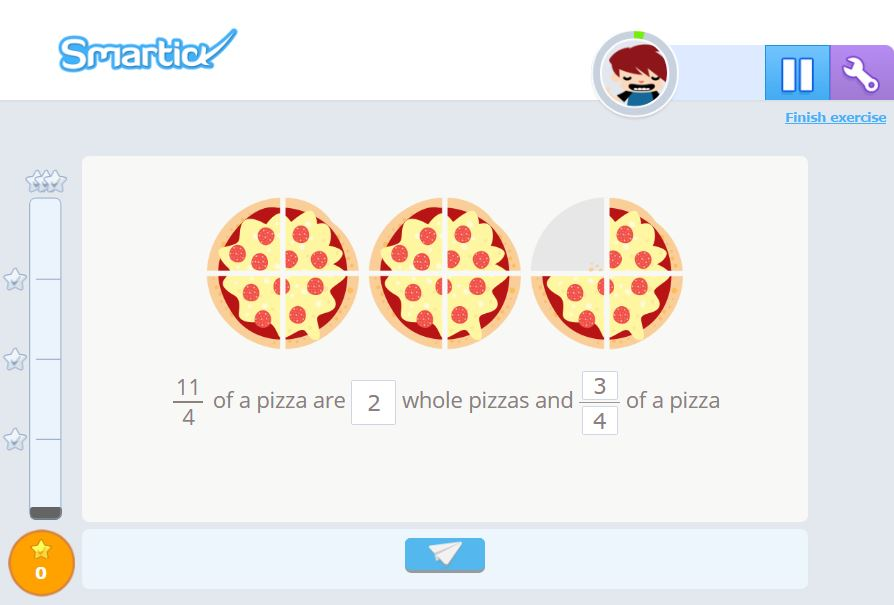This way it’s much easier to interpret, isn’t it?

### Example 3: Mixed numbers exercise

In the third type of exercise, we practice writing improper fractions and mixed numbers by representing them with circular charts. In the example, you can see 2 complete circles and 2 equal parts out of 3 of another.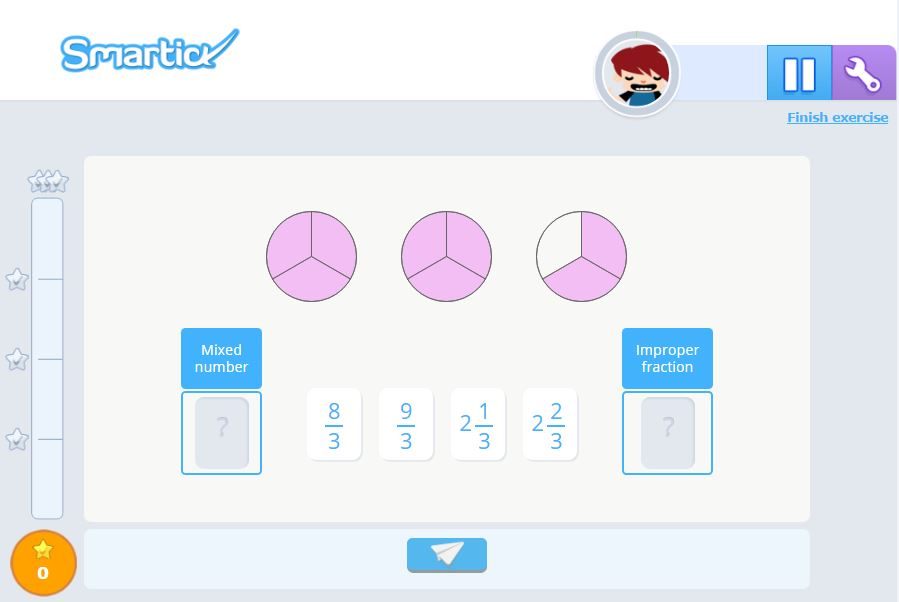Now the mixed number being represented is 223 and the improper fraction being represented is 83: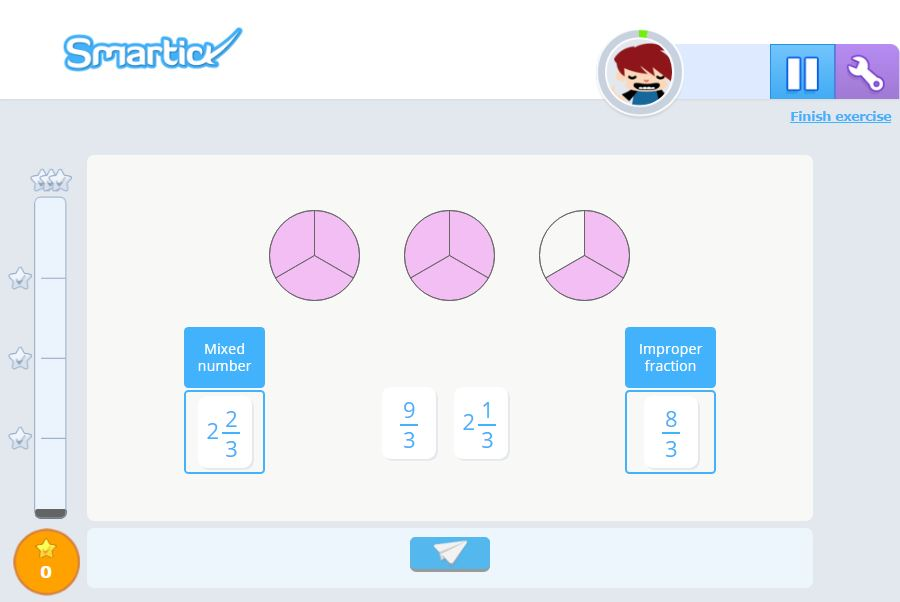### Example 4: Mixed numbers word problem

Finally, let’s look at an example from Smartick in which we work with mixed numbers to solve problems.Let’s calculate the number of laps Albie has to run around his planet. Since he has to run 12 more laps than Alan, and Alan has to run 910 of a lap, all we need to do is add 12 and 910.

Representing the result as a mixed number is really easy because you don’t even have to do anything to add 12 and 910, you just need to write the whole number followed by the fraction: 12910.Therefore we can say that Albie has to run 12910 laps around his planet.

And that’s all for today!

What do you think of this post? It’s really quick and easy to solve these exercises using mixed numbers, isn’t it?

• Maria Magdalena B. LohreNov 19 2020, 5:07 AM